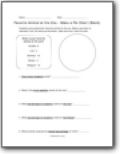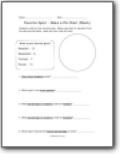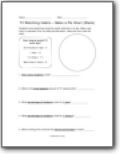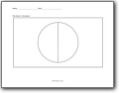# Pie (Circle) Graph Worksheets

### What is a Pie Graph?

Pie graph means writing a data in a circular graph and its slices indicate the data’s relative size. There is a kind of information’s pictorial representation. Categorical and numerical variables need to show its list in a pie chart. Pieces of a circular graph means chart’s parts and the word pie means the whole. We use this chart to address the numerical problems as it is the statistical graphic chart. If you want to know composition of something, using pie chart will be the best thing at that time. In particular circumstances, we will have to replace different graphs such as histograms, bar graph, and line plots. In case of pie charts, we need a specific section to track the total percentage as it consists of sectors and segments. The equal value of total data must be 360 degree and it always be 100%. For this, we have to implement the following steps to find out the percentage that is; Divide the details or data, Total calculations, Categorial division, Percentage conversion, and Degree calculation. According to it, the formula of the pie chart is given as the data/total value of data × 360 degree. Pie chart and its uses; In terms of business proceedings, there is a growth area. There is a categorical data representation.###### Favorite Animal at the Zoo

Students were asked their favorite animal at the zoo. Make a pie chart to represent from the data provide below. Label and color code the chart and then answer all the questions.###### Favorite Sport

See where class as a whole stands on all the different sports and where they want to go.###### TV Watching Habits

How long do watch TV each day?